# Valuations Aswath Damodaran 1 Companies Valued Company Model

• Slides: 74Valuations Aswath Damodaran 1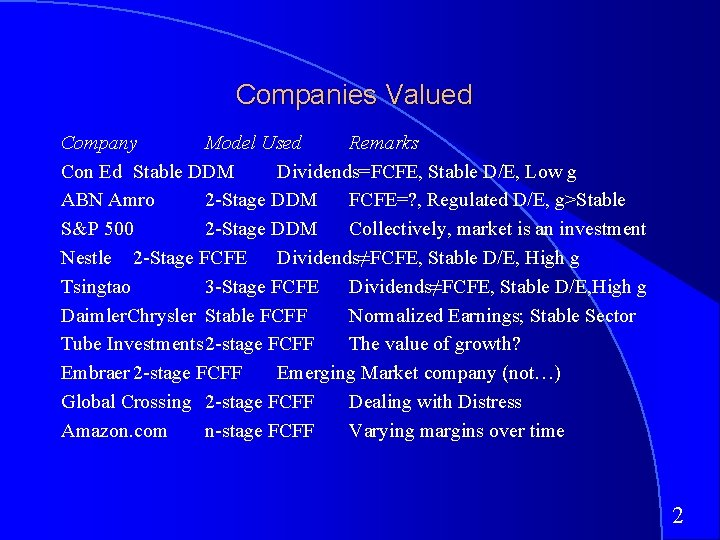Companies Valued Company Model Used Remarks Con Ed Stable DDM Dividends=FCFE, Stable D/E, Low g ABN Amro 2 -Stage DDM FCFE=? , Regulated D/E, g>Stable S&P 500 2 -Stage DDM Collectively, market is an investment Nestle 2 -Stage FCFE Dividends≠FCFE, Stable D/E, High g Tsingtao 3 -Stage FCFE Dividends≠FCFE, Stable D/E, High g Daimler. Chrysler Stable FCFF Normalized Earnings; Stable Sector Tube Investments 2 -stage FCFF The value of growth? Embraer 2 -stage FCFF Emerging Market company (not…) Global Crossing 2 -stage FCFF Dealing with Distress Amazon. com n-stage FCFF Varying margins over time 2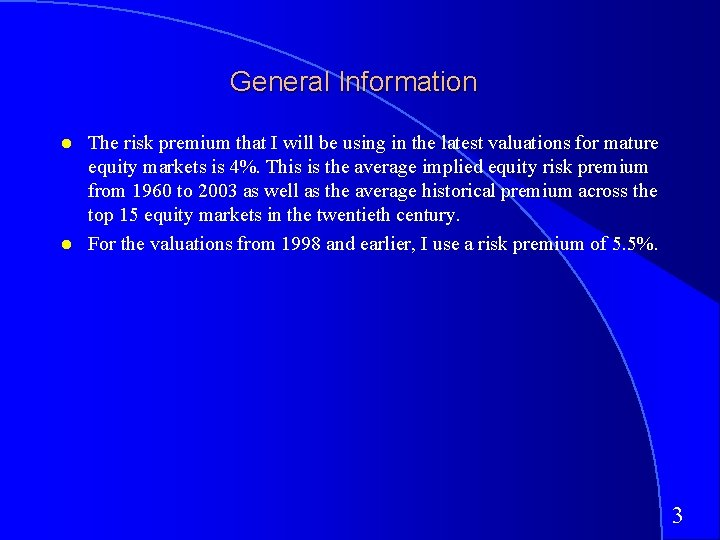General Information The risk premium that I will be using in the latest valuations for mature equity markets is 4%. This is the average implied equity risk premium from 1960 to 2003 as well as the average historical premium across the top 15 equity markets in the twentieth century. For the valuations from 1998 and earlier, I use a risk premium of 5. 5%. 3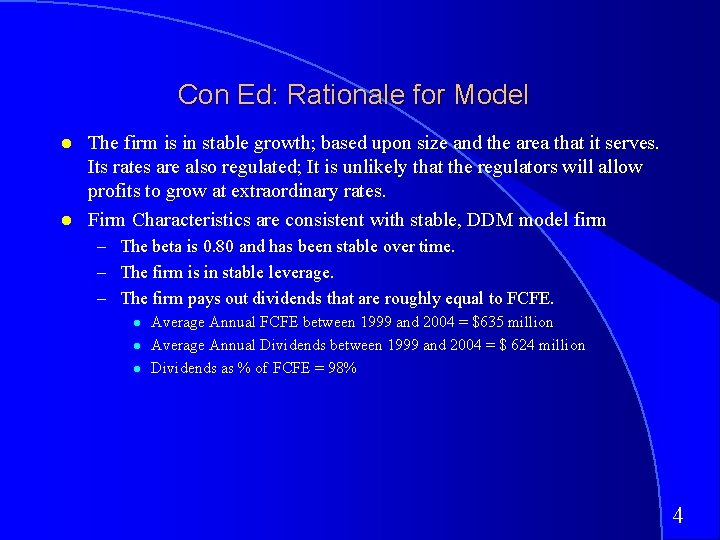Con Ed: Rationale for Model The firm is in stable growth; based upon size and the area that it serves. Its rates are also regulated; It is unlikely that the regulators will allow profits to grow at extraordinary rates. Firm Characteristics are consistent with stable, DDM model firm – The beta is 0. 80 and has been stable over time. – The firm is in stable leverage. – The firm pays out dividends that are roughly equal to FCFE. Average Annual FCFE between 1999 and 2004 = \$635 million Average Annual Dividends between 1999 and 2004 = \$ 624 million Dividends as % of FCFE = 98% 4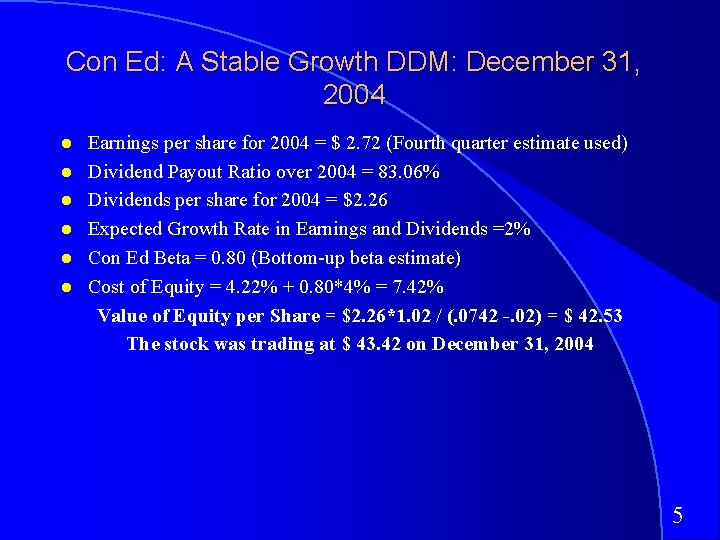Con Ed: A Stable Growth DDM: December 31, 2004 Earnings per share for 2004 = \$ 2. 72 (Fourth quarter estimate used) Dividend Payout Ratio over 2004 = 83. 06% Dividends per share for 2004 = \$2. 26 Expected Growth Rate in Earnings and Dividends =2% Con Ed Beta = 0. 80 (Bottom-up beta estimate) Cost of Equity = 4. 22% + 0. 80*4% = 7. 42% Value of Equity per Share = \$2. 26*1. 02 / (. 0742 -. 02) = \$ 42. 53 The stock was trading at \$ 43. 42 on December 31, 2004 5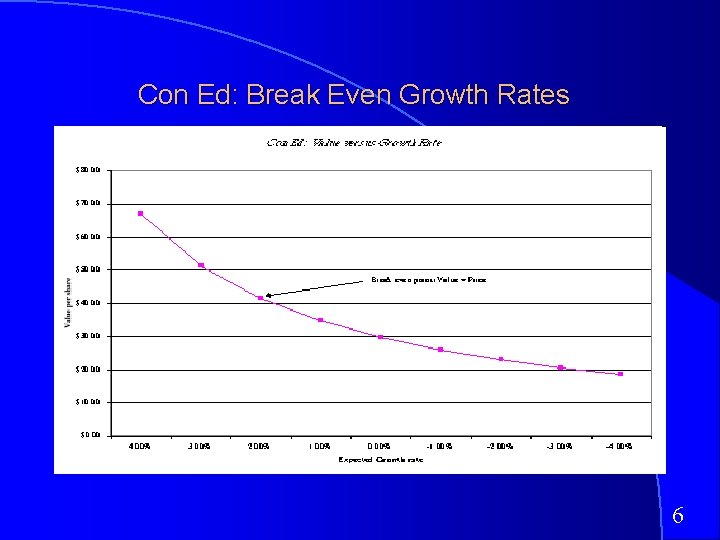Con Ed: Break Even Growth Rates 6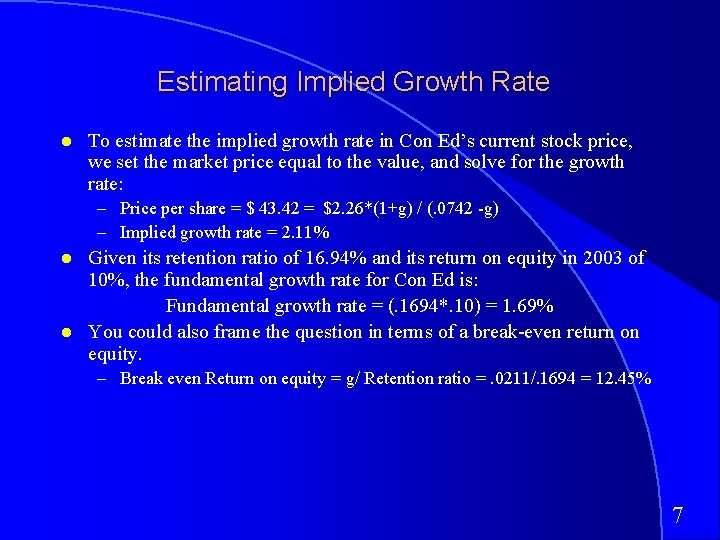Estimating Implied Growth Rate To estimate the implied growth rate in Con Ed’s current stock price, we set the market price equal to the value, and solve for the growth rate: – Price per share = \$ 43. 42 = \$2. 26*(1+g) / (. 0742 -g) – Implied growth rate = 2. 11% Given its retention ratio of 16. 94% and its return on equity in 2003 of 10%, the fundamental growth rate for Con Ed is: Fundamental growth rate = (. 1694*. 10) = 1. 69% You could also frame the question in terms of a break-even return on equity. – Break even Return on equity = g/ Retention ratio =. 0211/. 1694 = 12. 45% 7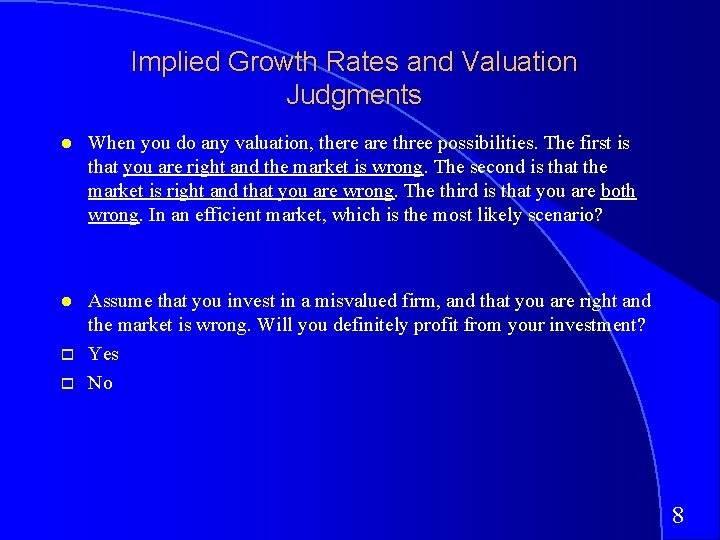Implied Growth Rates and Valuation Judgments When you do any valuation, there are three possibilities. The first is that you are right and the market is wrong. The second is that the market is right and that you are wrong. The third is that you are both wrong. In an efficient market, which is the most likely scenario? Assume that you invest in a misvalued firm, and that you are right and the market is wrong. Will you definitely profit from your investment? Yes No 8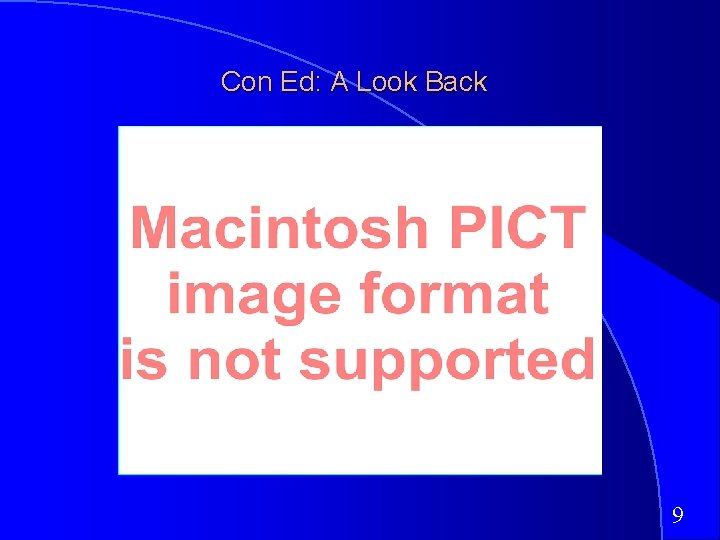Con Ed: A Look Back 9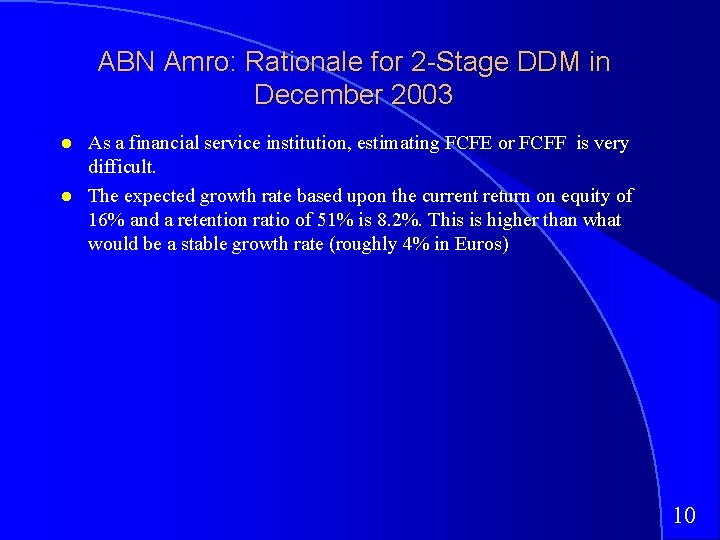ABN Amro: Rationale for 2 -Stage DDM in December 2003 As a financial service institution, estimating FCFE or FCFF is very difficult. The expected growth rate based upon the current return on equity of 16% and a retention ratio of 51% is 8. 2%. This is higher than what would be a stable growth rate (roughly 4% in Euros) 10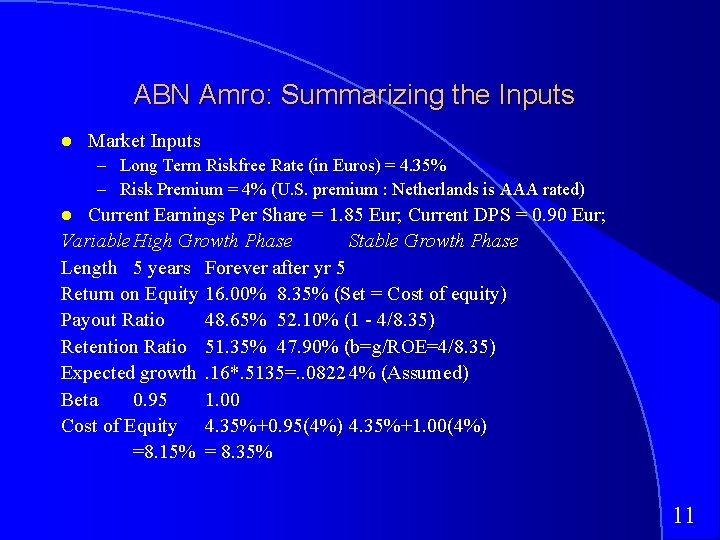ABN Amro: Summarizing the Inputs Market Inputs – Long Term Riskfree Rate (in Euros) = 4. 35% – Risk Premium = 4% (U. S. premium : Netherlands is AAA rated) Current Earnings Per Share = 1. 85 Eur; Current DPS = 0. 90 Eur; Variable High Growth Phase Stable Growth Phase Length 5 years Forever after yr 5 Return on Equity 16. 00% 8. 35% (Set = Cost of equity) Payout Ratio 48. 65% 52. 10% (1 - 4/8. 35) Retention Ratio 51. 35% 47. 90% (b=g/ROE=4/8. 35) Expected growth. 16*. 5135=. . 0822 4% (Assumed) Beta 0. 95 1. 00 Cost of Equity 4. 35%+0. 95(4%) 4. 35%+1. 00(4%) =8. 15% = 8. 35% 11ABN Amro: Valuation Year EPS DPS PV of DPS (at 8. 15%) 1 2. 00 0. 97 0. 90 2 2. 17 1. 05 0. 90 3 2. 34 1. 14 0. 90 4 2. 54 1. 23 0. 90 5 2. 75 1. 34 0. 90 Expected EPS in year 6 = 2. 75(1. 04) = 2. 86 Eur Expected DPS in year 6 = 2. 86*0. 5210=1. 49 Eur Terminal Price (in year 5) = 1. 49/(. 0835 -. 04) = 34. 20 Eur PV of Terminal Price = 34. 20/(1. 0815)5 = 23. 11 Eur Value Per Share = 0. 90 + 23. 11 = 27. 62 Eur The stock was trading at 18. 55 Euros on December 31, 2003 12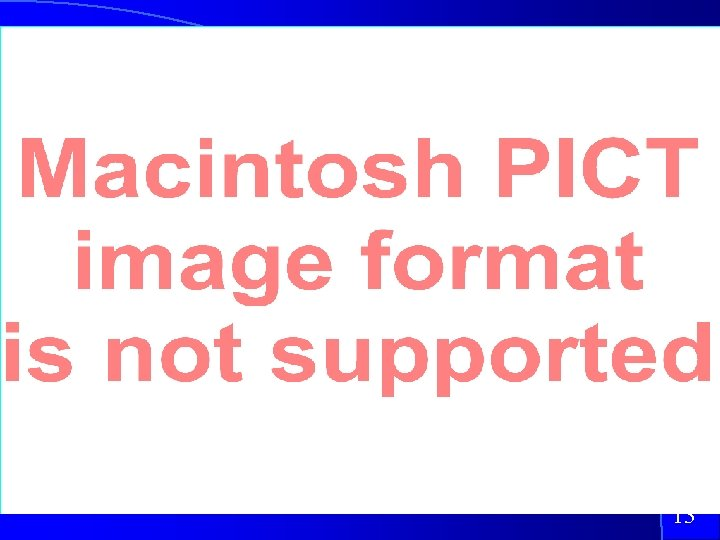13The Value of Growth In any valuation model, it is possible to extract the portion of the value that can be attributed to growth, and to break this down further into that portion attributable to “high growth” and the portion attributable to “stable growth”. In the case of the 2 -stage DDM, this can be accomplished as follows: Value of High Growth Value of Stable Assets in Growth Place DPSt = Expected dividends per share in year t r = Cost of Equity Pn = Price at the end of year n gn = Growth rate forever after year n 14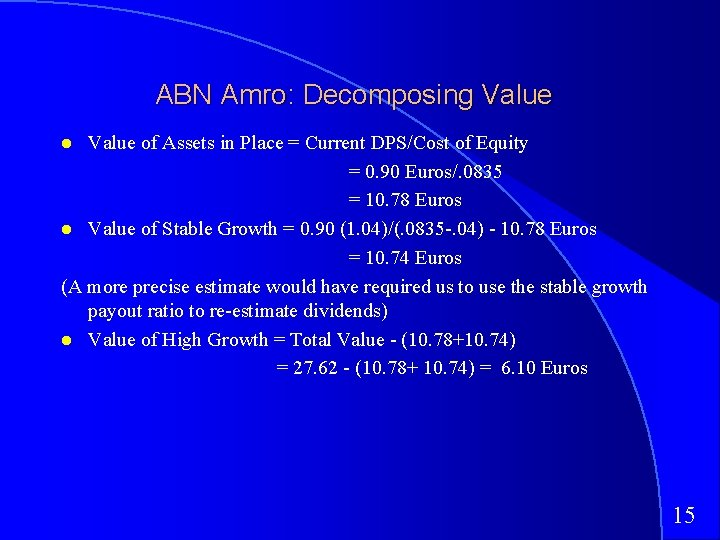ABN Amro: Decomposing Value of Assets in Place = Current DPS/Cost of Equity = 0. 90 Euros/. 0835 = 10. 78 Euros Value of Stable Growth = 0. 90 (1. 04)/(. 0835 -. 04) - 10. 78 Euros = 10. 74 Euros (A more precise estimate would have required us to use the stable growth payout ratio to re-estimate dividends) Value of High Growth = Total Value - (10. 78+10. 74) = 27. 62 - (10. 78+ 10. 74) = 6. 10 Euros 15S & P 500: Rationale for Use of Model While markets overall generally do not grow faster than the economies in which they operate, there is reason to believe that the earnings at U. S. companies (which have outpaced nominal GNP growth over the last 5 years) will continue to do so in the next 5 years. The consensus estimate of growth in earnings (from Zacks) is roughly 8% (with topdown estimates) Though it is possible to estimate FCFE for many of the firms in the S&P 500, it is not feasible for several (financial service firms). The dividends during the year should provide a reasonable (albeit conservative) estimate of the cash flows to equity investors from buying the index. 16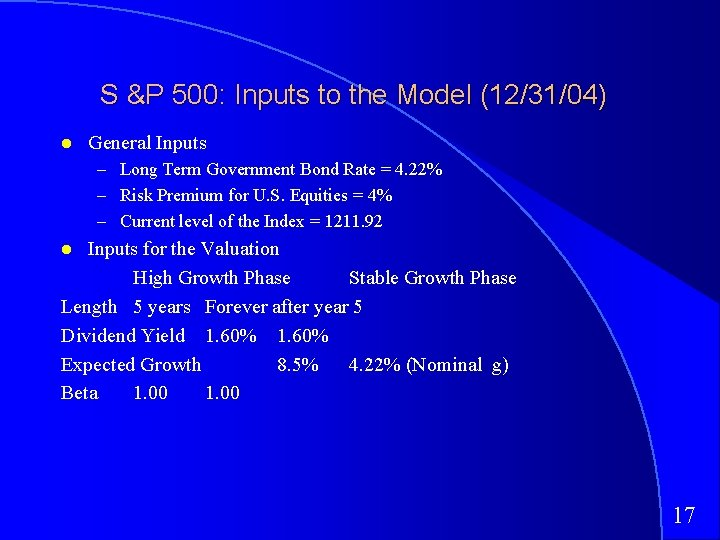S &P 500: Inputs to the Model (12/31/04) General Inputs – Long Term Government Bond Rate = 4. 22% – Risk Premium for U. S. Equities = 4% – Current level of the Index = 1211. 92 Inputs for the Valuation High Growth Phase Stable Growth Phase Length 5 years Forever after year 5 Dividend Yield 1. 60% Expected Growth 8. 5% 4. 22% (Nominal g) Beta 1. 00 17S & P 500: 2 -Stage DDM Valuation Cost of Equity = 4. 22% + 1(4%) = 8. 22% Terminal Value = 29. 18*1. 0422/(. 0822 -. 0422) = 760. 28 18Explaining the Difference The index is at 1212, while the model valuation comes in at 610. This indicates that one or more of the following has to be true. – The dividend discount model understates the value because dividends are less than FCFE. – The expected growth in earnings over the next 5 years will be much higher than 8%. – The risk premium used in the valuation (4%) is too high – The market is overvalued. 19A More Realistic Valuation of the Index We estimated the free cashflows to equity for each firm in the index and averaged the free cashflow to equity as a percent of market cap. The average FCFE yield for the index was about 2. 90% in 2004. With these inputs in the model: At a level of 1112, the market is overvalued by about 10%. 20Nestle: Rationale for Using Model - January 2001 Earnings per share at the firm has grown about 5% a year for the last 5 years, but the fundamentals at the firm suggest growth in EPS of about 11%. (Analysts are also forecasting a growth rate of 12% a year for the next 5 years) Nestle has a debt to capital ratio of about 37. 6% and is unlikely to change that leverage materially. (How do I know? I do not. I am just making an assumption. ) Like many large European firms, Nestle has paid less in dividends than it has available in FCFE. 21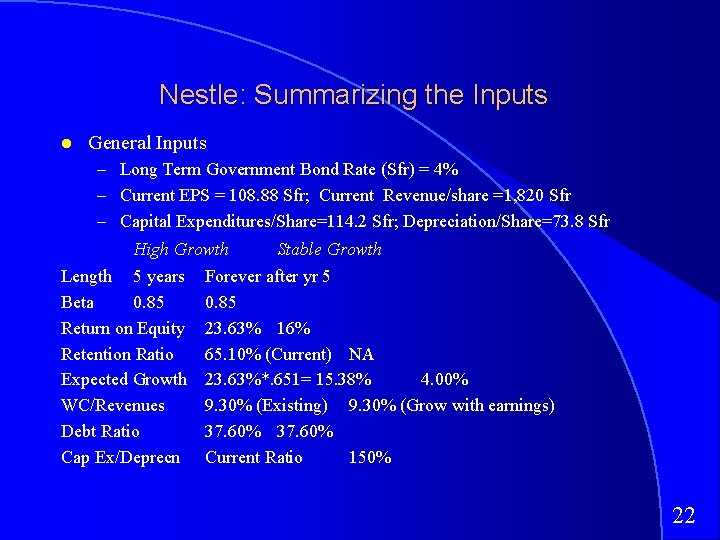Nestle: Summarizing the Inputs General Inputs – Long Term Government Bond Rate (Sfr) = 4% – Current EPS = 108. 88 Sfr; Current Revenue/share =1, 820 Sfr – Capital Expenditures/Share=114. 2 Sfr; Depreciation/Share=73. 8 Sfr High Growth Stable Growth Length 5 years Forever after yr 5 Beta 0. 85 Return on Equity 23. 63% 16% Retention Ratio 65. 10% (Current) NA Expected Growth 23. 63%*. 651= 15. 38% 4. 00% WC/Revenues 9. 30% (Existing) 9. 30% (Grow with earnings) Debt Ratio 37. 60% Cap Ex/Deprecn Current Ratio 150% 22Estimating the Risk Premium for Nestle Revenues Weight Risk Premium North America 17. 5 24. 82% 4. 00% South America 4. 3 6. 10% 12. 00% Switzerland 1. 1 1. 56% 4. 00% Germany/France/UK 18. 4 26. 10% 4. 00% Italy/Spain 6. 4 9. 08% 5. 50% Asia 5. 8 8. 23% 9. 00% Rest of W. Europe 13 18. 44% 4. 00% Eastern Europe 4 5. 67% 8. 00% Total 70. 5 100. 00% 5. 26% The risk premium that we will use in the valuation is 5. 26% Cost of Equity = 4% + 0. 85 (5. 26%) = 8. 47% 23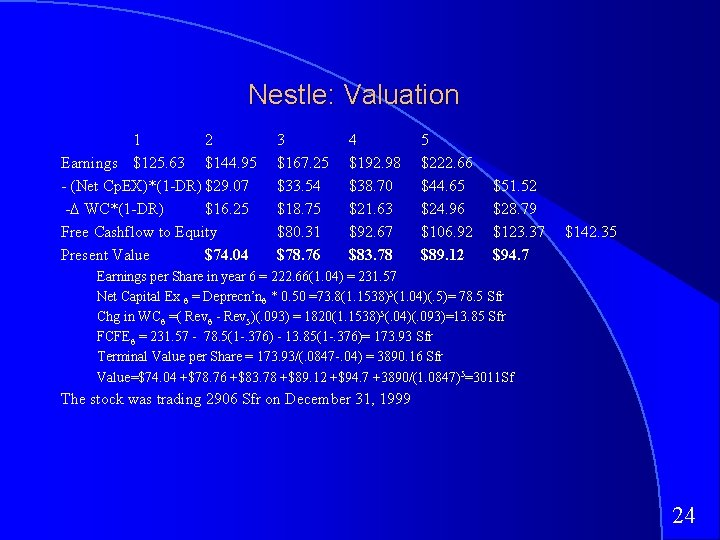Nestle: Valuation 1 2 Earnings \$125. 63 \$144. 95 - (Net Cp. EX)*(1 -DR) \$29. 07 -D WC*(1 -DR) \$16. 25 Free Cashflow to Equity Present Value \$74. 04 3 \$167. 25 \$33. 54 \$18. 75 \$80. 31 \$78. 76 4 \$192. 98 \$38. 70 \$21. 63 \$92. 67 \$83. 78 5 \$222. 66 \$44. 65 \$24. 96 \$106. 92 \$89. 12 \$51. 52 \$28. 79 \$123. 37 \$94. 7 \$142. 35 Earnings per Share in year 6 = 222. 66(1. 04) = 231. 57 Net Capital Ex 6 = Deprecn’n 6 * 0. 50 =73. 8(1. 1538)5(1. 04)(. 5)= 78. 5 Sfr Chg in WC 6 =( Rev 6 - Rev 5)(. 093) = 1820(1. 1538)5(. 04)(. 093)=13. 85 Sfr FCFE 6 = 231. 57 - 78. 5(1 -. 376) - 13. 85(1 -. 376)= 173. 93 Sfr Terminal Value per Share = 173. 93/(. 0847 -. 04) = 3890. 16 Sfr Value=\$74. 04 +\$78. 76 +\$83. 78 +\$89. 12 +\$94. 7 +3890/(1. 0847)5=3011 Sf The stock was trading 2906 Sfr on December 31, 1999 24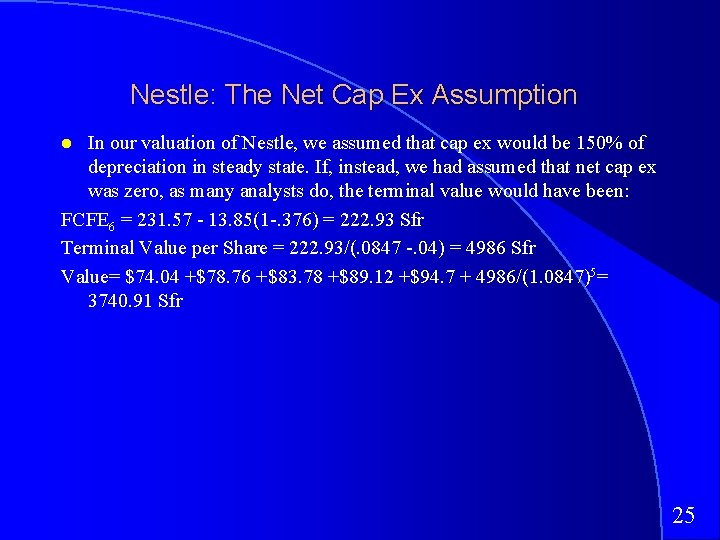Nestle: The Net Cap Ex Assumption In our valuation of Nestle, we assumed that cap ex would be 150% of depreciation in steady state. If, instead, we had assumed that net cap ex was zero, as many analysts do, the terminal value would have been: FCFE 6 = 231. 57 - 13. 85(1 -. 376) = 222. 93 Sfr Terminal Value per Share = 222. 93/(. 0847 -. 04) = 4986 Sfr Value= \$74. 04 +\$78. 76 +\$83. 78 +\$89. 12 +\$94. 7 + 4986/(1. 0847)5= 3740. 91 Sfr 25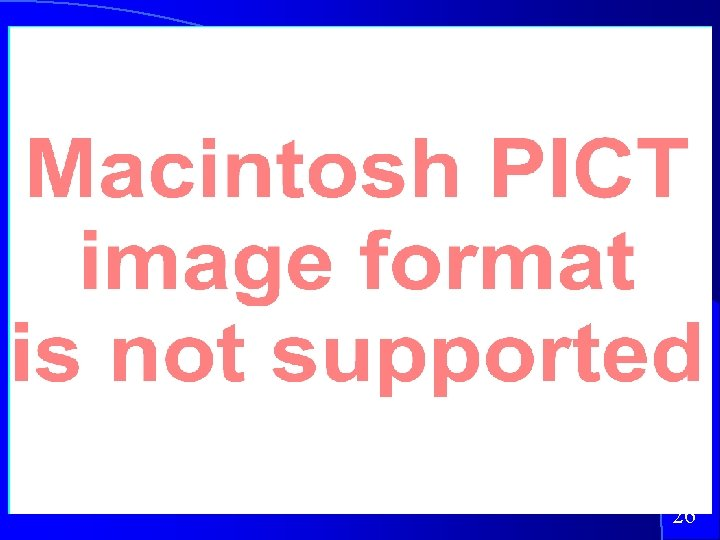26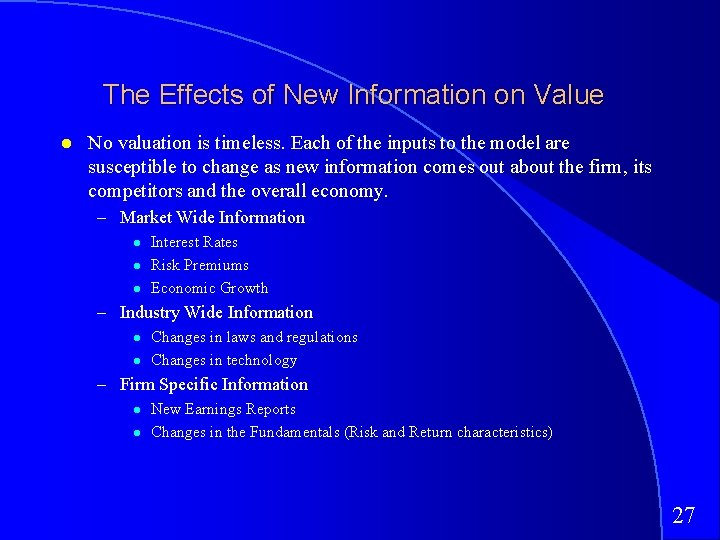The Effects of New Information on Value No valuation is timeless. Each of the inputs to the model are susceptible to change as new information comes out about the firm, its competitors and the overall economy. – Market Wide Information Interest Rates Risk Premiums Economic Growth – Industry Wide Information Changes in laws and regulations Changes in technology – Firm Specific Information New Earnings Reports Changes in the Fundamentals (Risk and Return characteristics) 27Nestle: Effects of an Earnings Announcement Assume that Nestle makes an earnings announcement which includes two pieces of news: – The earnings per share come in lower than expected. The base year earnings per share will be 105. 5 Sfr instead of 108. 8 Sfr. – Increased competition in its markets is putting downward pressure on the net profit margin. The after-tax margin, which was 5. 98% in the previous analysis, is expected to shrink to 5. 79%. There are two effects on value: – The drop in earnings will make the projected earnings and cash flows lower, even if the growth rate remains the same – The drop in net margin will make the return on equity lower (assuming turnover ratios remain unchanged). This will reduce expected growth. 2829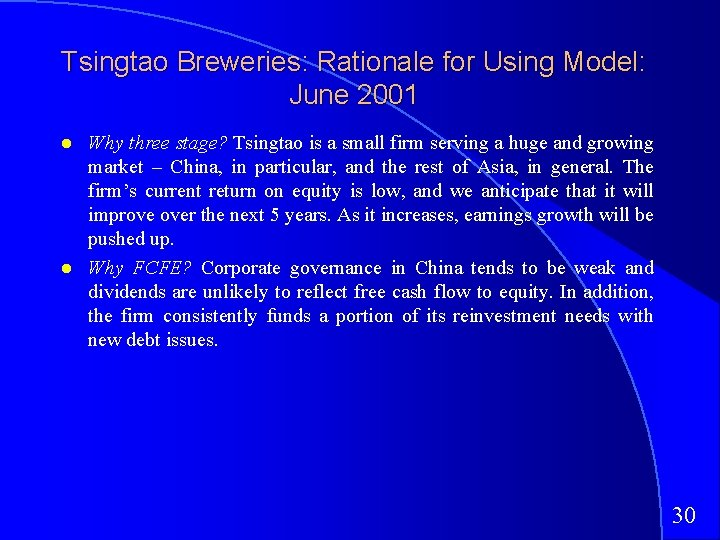Tsingtao Breweries: Rationale for Using Model: June 2001 Why three stage? Tsingtao is a small firm serving a huge and growing market – China, in particular, and the rest of Asia, in general. The firm’s current return on equity is low, and we anticipate that it will improve over the next 5 years. As it increases, earnings growth will be pushed up. Why FCFE? Corporate governance in China tends to be weak and dividends are unlikely to reflect free cash flow to equity. In addition, the firm consistently funds a portion of its reinvestment needs with new debt issues. 30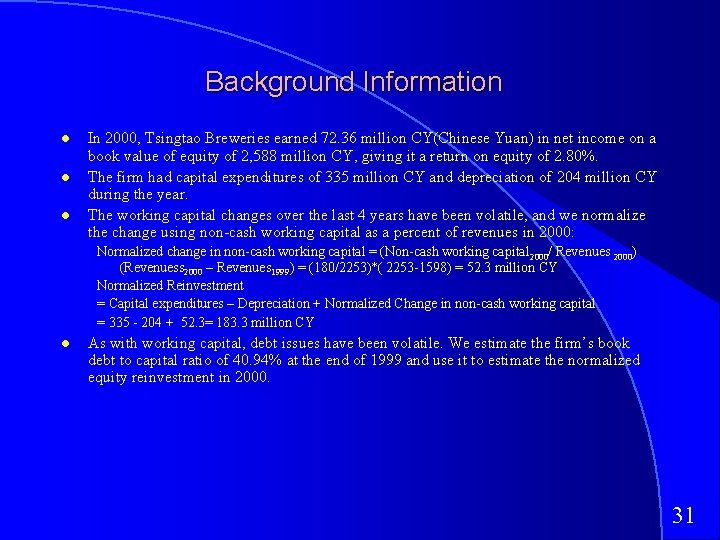Background Information In 2000, Tsingtao Breweries earned 72. 36 million CY(Chinese Yuan) in net income on a book value of equity of 2, 588 million CY, giving it a return on equity of 2. 80%. The firm had capital expenditures of 335 million CY and depreciation of 204 million CY during the year. The working capital changes over the last 4 years have been volatile, and we normalize the change using non-cash working capital as a percent of revenues in 2000: Normalized change in non-cash working capital = (Non-cash working capital 2000/ Revenues 2000) (Revenuess 2000 – Revenues 1999) = (180/2253)*( 2253 -1598) = 52. 3 million CY Normalized Reinvestment = Capital expenditures – Depreciation + Normalized Change in non-cash working capital = 335 - 204 + 52. 3= 183. 3 million CY As with working capital, debt issues have been volatile. We estimate the firm’s book debt to capital ratio of 40. 94% at the end of 1999 and use it to estimate the normalized equity reinvestment in 2000. 31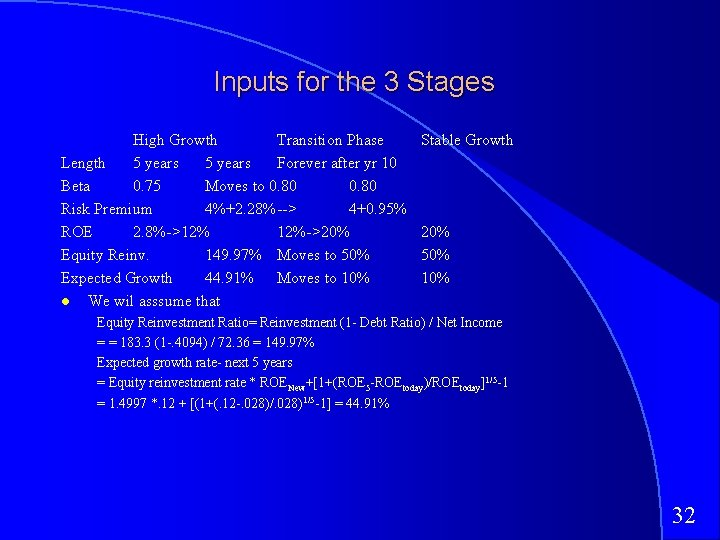Inputs for the 3 Stages High Growth Transition Phase Length 5 years Forever after yr 10 Beta 0. 75 Moves to 0. 80 Risk Premium 4%+2. 28%--> 4+0. 95% ROE 2. 8%->12% 12%->20% Equity Reinv. 149. 97% Moves to 50% Expected Growth 44. 91% Moves to 10% We wil asssume that Stable Growth 20% 50% 10% Equity Reinvestment Ratio= Reinvestment (1 - Debt Ratio) / Net Income = = 183. 3 (1 -. 4094) / 72. 36 = 149. 97% Expected growth rate- next 5 years = Equity reinvestment rate * ROENew+[1+(ROE 5 -ROEtoday)/ROEtoday]1/5 -1 = 1. 4997 *. 12 + [(1+(. 12 -. 028)/. 028)1/5 -1] = 44. 91% 32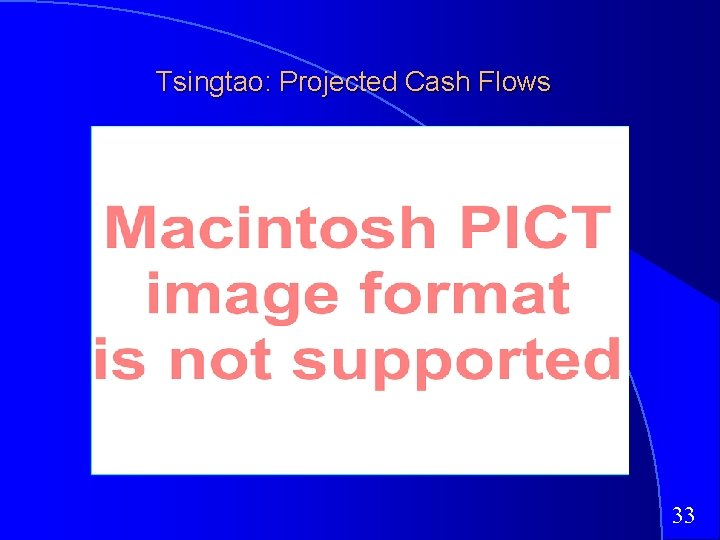Tsingtao: Projected Cash Flows 33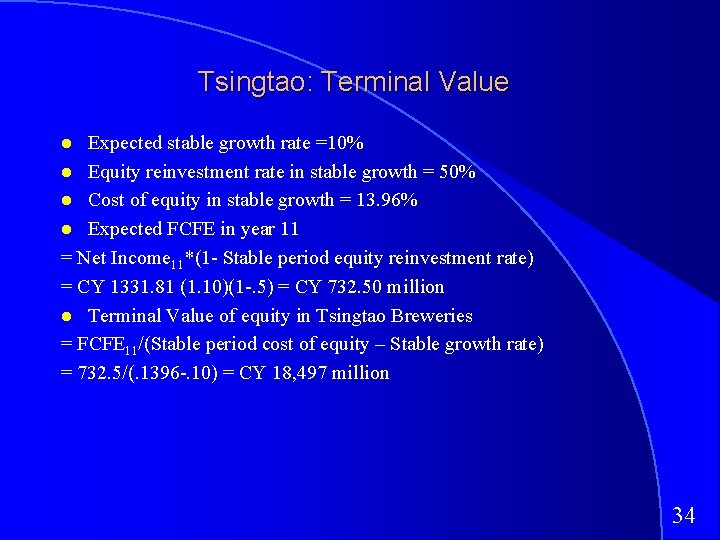Tsingtao: Terminal Value Expected stable growth rate =10% Equity reinvestment rate in stable growth = 50% Cost of equity in stable growth = 13. 96% Expected FCFE in year 11 = Net Income 11*(1 - Stable period equity reinvestment rate) = CY 1331. 81 (1. 10)(1 -. 5) = CY 732. 50 million Terminal Value of equity in Tsingtao Breweries = FCFE 11/(Stable period cost of equity – Stable growth rate) = 732. 5/(. 1396 -. 10) = CY 18, 497 million 34Tsingtao: Valuation Value of Equity = PV of FCFE during the high growth period + PV of terminal value =-CY 186. 65+CY 18, 497/(1. 14715*1. 1456*1. 1441*1. 1426*1. 1411*1. 1396) = CY 4, 596 million Value of Equity per share = Value of Equity/ Number of Shares = CY 4, 596/653. 15 = CY 7. 04 per share The stock was trading at 10. 10 Yuan per share, which would make it overvalued, based upon this valuation. 35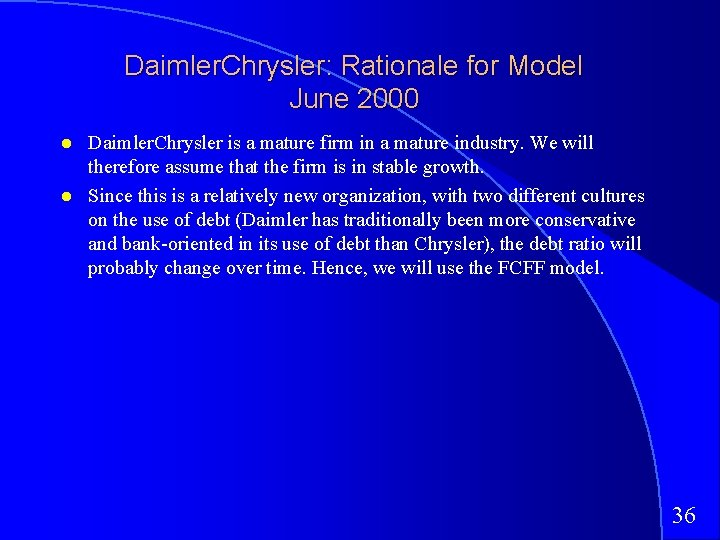Daimler. Chrysler: Rationale for Model June 2000 Daimler. Chrysler is a mature firm in a mature industry. We will therefore assume that the firm is in stable growth. Since this is a relatively new organization, with two different cultures on the use of debt (Daimler has traditionally been more conservative and bank-oriented in its use of debt than Chrysler), the debt ratio will probably change over time. Hence, we will use the FCFF model. 36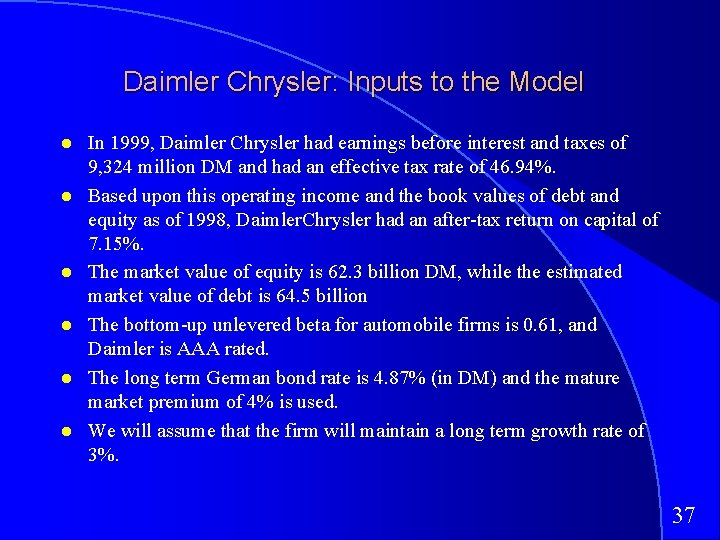Daimler Chrysler: Inputs to the Model In 1999, Daimler Chrysler had earnings before interest and taxes of 9, 324 million DM and had an effective tax rate of 46. 94%. Based upon this operating income and the book values of debt and equity as of 1998, Daimler. Chrysler had an after-tax return on capital of 7. 15%. The market value of equity is 62. 3 billion DM, while the estimated market value of debt is 64. 5 billion The bottom-up unlevered beta for automobile firms is 0. 61, and Daimler is AAA rated. The long term German bond rate is 4. 87% (in DM) and the mature market premium of 4% is used. We will assume that the firm will maintain a long term growth rate of 3%. 37Daimler/Chrysler: Analyzing the Inputs Expected Reinvestment Rate = g/ ROC = 3%/7. 15% = 41. 98% Cost of Capital – – Bottom-up Levered Beta = 0. 61 (1+(1 -. 4694)(64. 5/62. 3)) = 0. 945 Cost of Equity = 4. 87% + 0. 945 (4%) = 8. 65% After-tax Cost of Debt = (4. 87% + 0. 20%) (1 -. 4694)= 2. 69% Cost of Capital = 8. 65%(62. 3/(62. 3+64. 5))+ 2. 69% (64. 5/(62. 3+64. 5)) = 5. 62% 38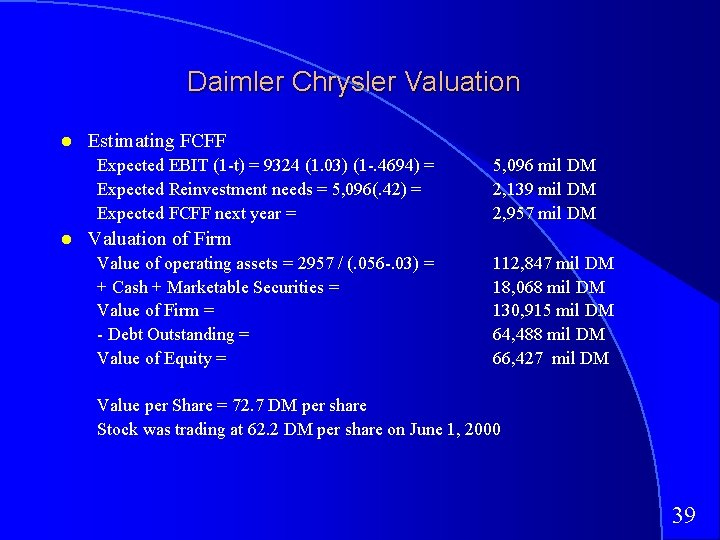Daimler Chrysler Valuation Estimating FCFF Expected EBIT (1 -t) = 9324 (1. 03) (1 -. 4694) = Expected Reinvestment needs = 5, 096(. 42) = Expected FCFF next year = 5, 096 mil DM 2, 139 mil DM 2, 957 mil DM Valuation of Firm Value of operating assets = 2957 / (. 056 -. 03) = + Cash + Marketable Securities = Value of Firm = - Debt Outstanding = Value of Equity = 112, 847 mil DM 18, 068 mil DM 130, 915 mil DM 64, 488 mil DM 66, 427 mil DM Value per Share = 72. 7 DM per share Stock was trading at 62. 2 DM per share on June 1, 2000 39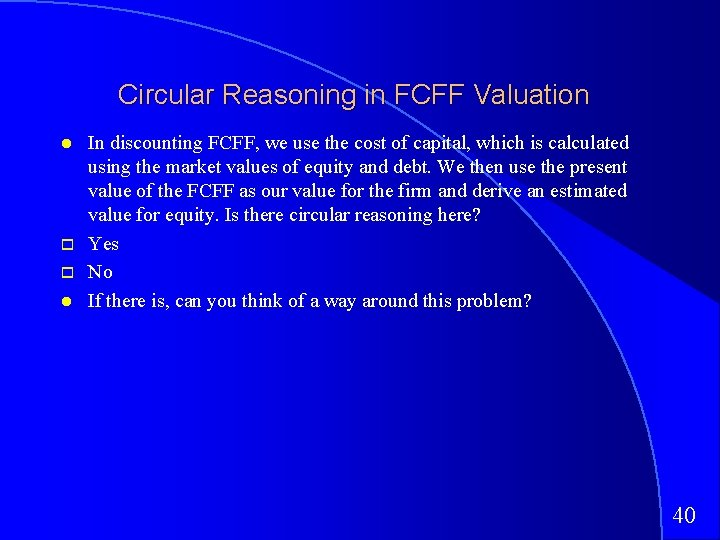Circular Reasoning in FCFF Valuation In discounting FCFF, we use the cost of capital, which is calculated using the market values of equity and debt. We then use the present value of the FCFF as our value for the firm and derive an estimated value for equity. Is there circular reasoning here? Yes No If there is, can you think of a way around this problem? 40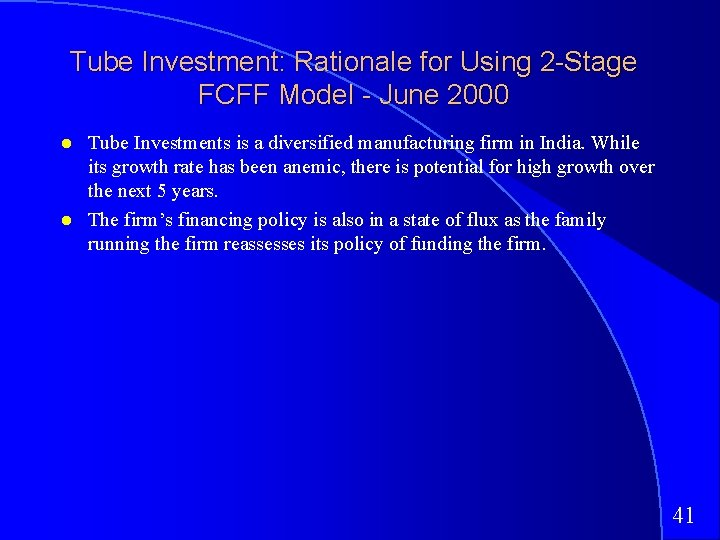Tube Investment: Rationale for Using 2 -Stage FCFF Model - June 2000 Tube Investments is a diversified manufacturing firm in India. While its growth rate has been anemic, there is potential for high growth over the next 5 years. The firm’s financing policy is also in a state of flux as the family running the firm reassesses its policy of funding the firm. 4142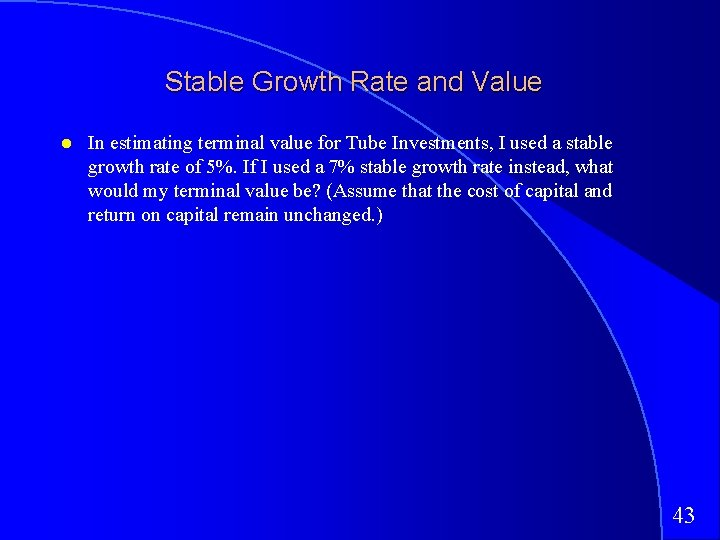Stable Growth Rate and Value In estimating terminal value for Tube Investments, I used a stable growth rate of 5%. If I used a 7% stable growth rate instead, what would my terminal value be? (Assume that the cost of capital and return on capital remain unchanged. ) 43The Effects of Return Improvements on Value The firm is considering changes in the way in which it invests, which management believes will increase the return on capital to 12. 20% on just new investments (and not on existing investments) over the next 5 years. The value of the firm will be higher, because of higher expected growth. 4445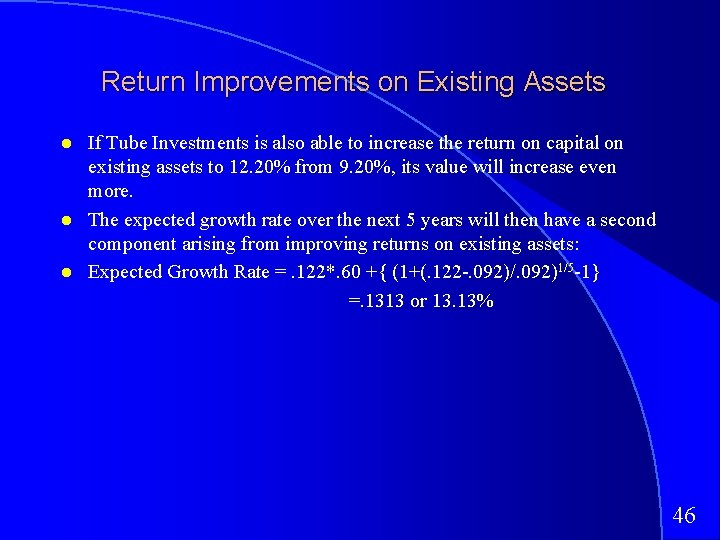Return Improvements on Existing Assets If Tube Investments is also able to increase the return on capital on existing assets to 12. 20% from 9. 20%, its value will increase even more. The expected growth rate over the next 5 years will then have a second component arising from improving returns on existing assets: Expected Growth Rate =. 122*. 60 +{ (1+(. 122 -. 092)/. 092)1/5 -1} =. 1313 or 13. 13% 4647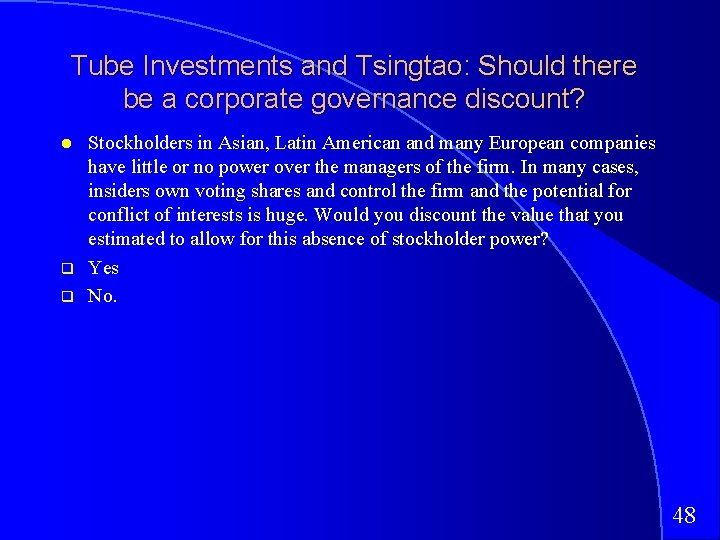Tube Investments and Tsingtao: Should there be a corporate governance discount? q q Stockholders in Asian, Latin American and many European companies have little or no power over the managers of the firm. In many cases, insiders own voting shares and control the firm and the potential for conflict of interests is huge. Would you discount the value that you estimated to allow for this absence of stockholder power? Yes No. 48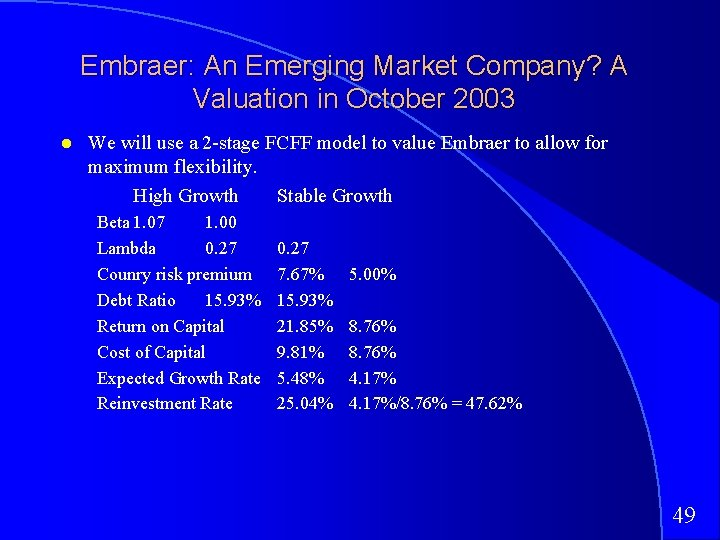Embraer: An Emerging Market Company? A Valuation in October 2003 We will use a 2 -stage FCFF model to value Embraer to allow for maximum flexibility. High Growth Stable Growth Beta 1. 07 1. 00 Lambda 0. 27 Counry risk premium Debt Ratio 15. 93% Return on Capital Cost of Capital Expected Growth Rate Reinvestment Rate 0. 27 7. 67% 15. 93% 21. 85% 9. 81% 5. 48% 25. 04% 5. 00% 8. 76% 4. 17%/8. 76% = 47. 62% 49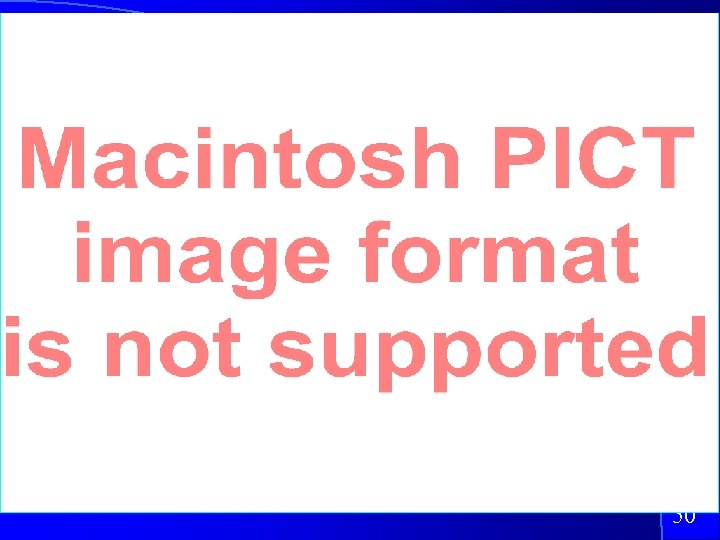50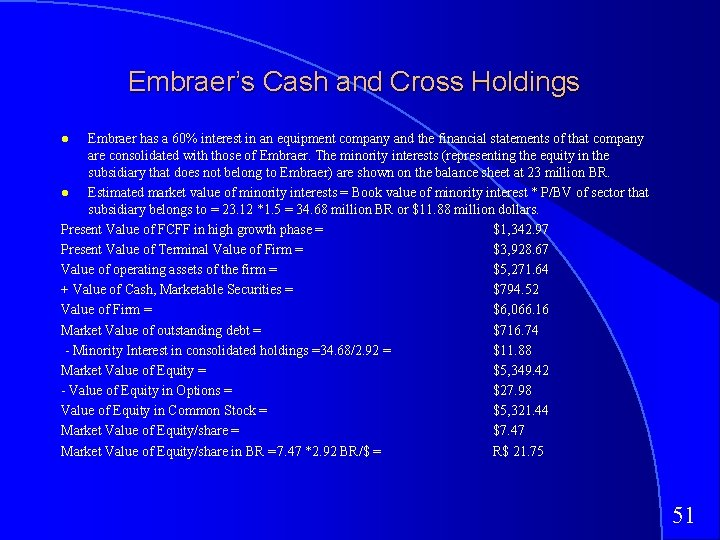Embraer’s Cash and Cross Holdings Embraer has a 60% interest in an equipment company and the financial statements of that company are consolidated with those of Embraer. The minority interests (representing the equity in the subsidiary that does not belong to Embraer) are shown on the balance sheet at 23 million BR. Estimated market value of minority interests = Book value of minority interest * P/BV of sector that subsidiary belongs to = 23. 12 *1. 5 = 34. 68 million BR or \$11. 88 million dollars. Present Value of FCFF in high growth phase = \$1, 342. 97 Present Value of Terminal Value of Firm = \$3, 928. 67 Value of operating assets of the firm = \$5, 271. 64 + Value of Cash, Marketable Securities = \$794. 52 Value of Firm = \$6, 066. 16 Market Value of outstanding debt = \$716. 74 - Minority Interest in consolidated holdings =34. 68/2. 92 = \$11. 88 Market Value of Equity = \$5, 349. 42 - Value of Equity in Options = \$27. 98 Value of Equity in Common Stock = \$5, 321. 44 Market Value of Equity/share = \$7. 47 Market Value of Equity/share in BR = 7. 47 *2. 92 BR/\$ = R\$ 21. 75 51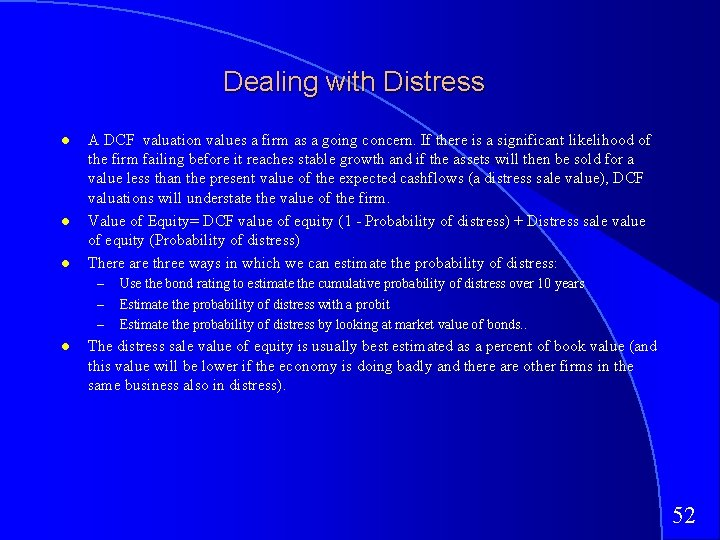Dealing with Distress A DCF valuation values a firm as a going concern. If there is a significant likelihood of the firm failing before it reaches stable growth and if the assets will then be sold for a value less than the present value of the expected cashflows (a distress sale value), DCF valuations will understate the value of the firm. Value of Equity= DCF value of equity (1 - Probability of distress) + Distress sale value of equity (Probability of distress) There are three ways in which we can estimate the probability of distress: – – – Use the bond rating to estimate the cumulative probability of distress over 10 years Estimate the probability of distress with a probit Estimate the probability of distress by looking at market value of bonds. . The distress sale value of equity is usually best estimated as a percent of book value (and this value will be lower if the economy is doing badly and there are other firms in the same business also in distress). 5253Valuing Global Crossing with Distress Probability of distress – Price of 8 year, 12% bond issued by Global Crossing = \$ 653 – Probability of distress = 13. 53% a year – Cumulative probability of survival over 10 years = (1 -. 1353)10 = 23. 37% Distress sale value of equity – – Book value of capital = \$14, 531 million Distress sale value = 15% of book value =. 15*14531 = \$2, 180 million Book value of debt = \$7, 647 million Distress sale value of equity = \$ 0 Distress adjusted value of equity – Value of Global Crossing = \$3. 22 (. 2337) + \$0. 00 (. 7663) = \$0. 75 54The Dark Side of Valuation Aswath Damodaran http: //www. stern. nyu. edu/~adamodar 55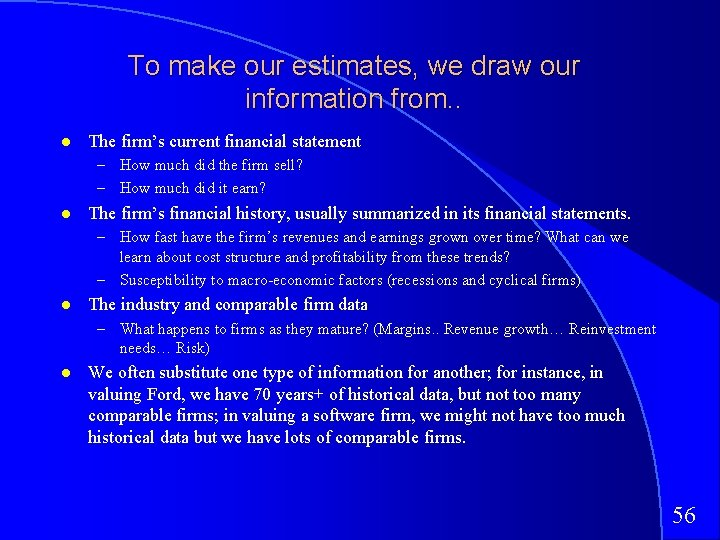To make our estimates, we draw our information from. . The firm’s current financial statement – How much did the firm sell? – How much did it earn? The firm’s financial history, usually summarized in its financial statements. – How fast have the firm’s revenues and earnings grown over time? What can we learn about cost structure and profitability from these trends? – Susceptibility to macro-economic factors (recessions and cyclical firms) The industry and comparable firm data – What happens to firms as they mature? (Margins. . Revenue growth… Reinvestment needs… Risk) We often substitute one type of information for another; for instance, in valuing Ford, we have 70 years+ of historical data, but not too many comparable firms; in valuing a software firm, we might not have too much historical data but we have lots of comparable firms. 56The Dark Side. . . Valuation is most difficult when a company – Has negative earnings and low revenues in its current financial statements – No history – No comparables ( or even if they exist, they are all at the same stage of the life cycle as the firm being valued) 5758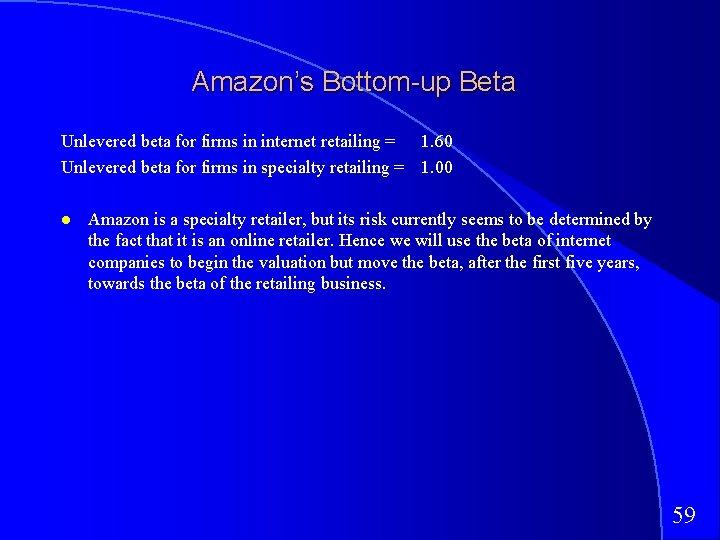Amazon’s Bottom-up Beta Unlevered beta for firms in internet retailing = 1. 60 Unlevered beta for firms in specialty retailing = 1. 00 Amazon is a specialty retailer, but its risk currently seems to be determined by the fact that it is an online retailer. Hence we will use the beta of internet companies to begin the valuation but move the beta, after the first five years, towards the beta of the retailing business. 59Estimating Synthetic Ratings and cost of debt The rating for a firm can be estimated using the financial characteristics of the firm. In its simplest form, the rating can be estimated from the interest coverage ratio Interest Coverage Ratio = EBIT / Interest Expenses Amazon. com has negative operating income; this yields a negative interest coverage ratio, which should suggest a low rating. We computed an average interest coverage ratio of 2. 82 over the next 5 years. This yields an average rating of BBB for Amazon. com for the first 5 years. (In effect, the rating will be lower in the earlier years and higher in the later years than BBB) 60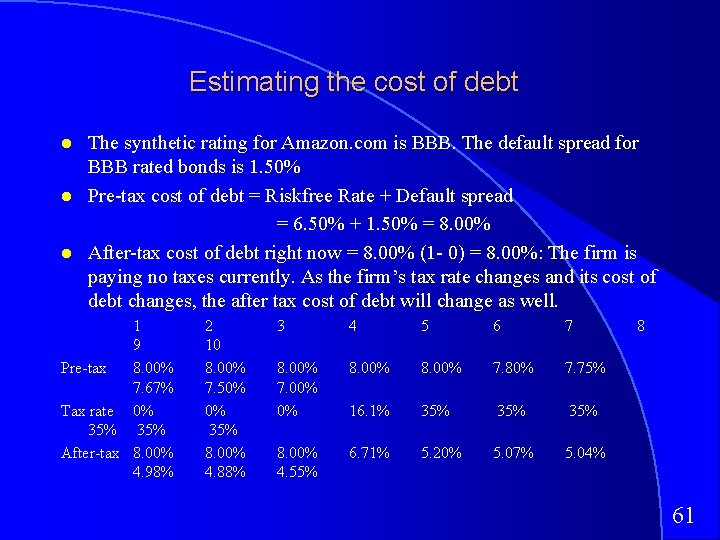Estimating the cost of debt The synthetic rating for Amazon. com is BBB. The default spread for BBB rated bonds is 1. 50% Pre-tax cost of debt = Riskfree Rate + Default spread = 6. 50% + 1. 50% = 8. 00% After-tax cost of debt right now = 8. 00% (1 - 0) = 8. 00%: The firm is paying no taxes currently. As the firm’s tax rate changes and its cost of debt changes, the after tax cost of debt will change as well. 1 9 Pre-tax 8. 00% 7. 67% Tax rate 0% 35% After-tax 8. 00% 4. 98% 2 10 8. 00% 7. 50% 0% 35% 8. 00% 4. 88% 3 4 5 6 7 8. 00% 7. 00% 0% 8. 00% 7. 80% 7. 75% 16. 1% 35% 35% 8. 00% 4. 55% 6. 71% 5. 20% 5. 07% 5. 04% 8 61Estimating Cost of Capital: Amazon. com Equity – Cost of Equity = 6. 50% + 1. 60 (4. 00%) = 12. 90% – Market Value of Equity = \$ 84/share* 340. 79 mil shs = \$ 28, 626 mil (98. 8%) Debt – Cost of debt = 6. 50% + 1. 50% (default spread) = 8. 00% – Market Value of Debt = \$ 349 mil (1. 2%) Cost of Capital = 12. 9 % (. 988) + 8. 00% (1 - 0) (. 012)) = 12. 84% Amazon. com has a book value of equity of \$ 138 million and a book value of debt of \$ 349 million. Shows you how irrelevant book value is in this process. 62Calendar Years, Financial Years and Updated Information The operating income and revenue that we use in valuation should be updated numbers. One of the problems with using financial statements is that they are dated. As a general rule, it is better to use 12 -month trailing estimates for earnings and revenues than numbers for the most recent financial year. This rule becomes even more critical when valuing companies that are evolving and growing rapidly. Last 10 -K Trailing 12 -month Revenues \$ 610 million \$1, 117 million EBIT - \$125 million - \$ 410 million 63Are S, G & A expenses capital expenditures? Many internet companies are arguing that selling and G&A expenses are the equivalent of R&D expenses for a high-technology firms and should be treated as capital expenditures. If we adopt this rationale, we should be computing earnings before these expenses, which will make many of these firms profitable. It will also mean that they are reinvesting far more than we think they are. It will, however, make not their cash flows less negative. Should Amazon. com’s selling expenses be treated as cap ex? 64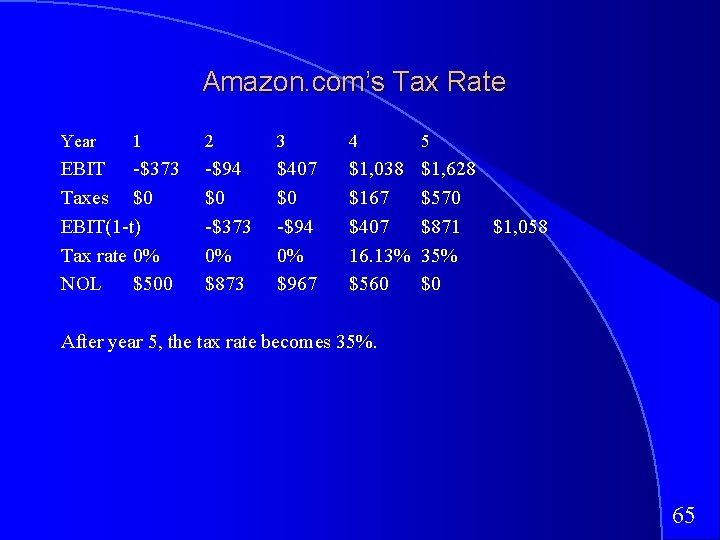Amazon. com’s Tax Rate Year 1 EBIT -\$373 Taxes \$0 EBIT(1 -t) Tax rate 0% NOL \$500 2 3 4 5 -\$94 \$0 -\$373 0% \$873 \$407 \$0 -\$94 0% \$967 \$1, 038 \$167 \$407 16. 13% \$560 \$1, 628 \$570 \$871 \$1, 058 35% \$0 After year 5, the tax rate becomes 35%. 65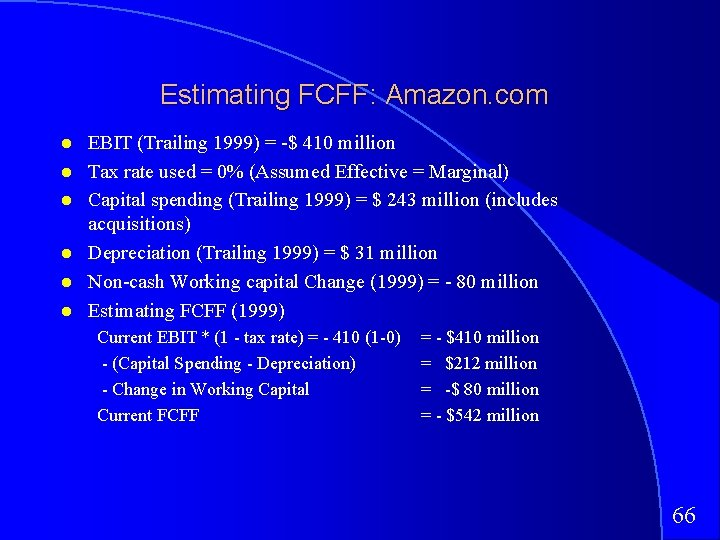Estimating FCFF: Amazon. com EBIT (Trailing 1999) = -\$ 410 million Tax rate used = 0% (Assumed Effective = Marginal) Capital spending (Trailing 1999) = \$ 243 million (includes acquisitions) Depreciation (Trailing 1999) = \$ 31 million Non-cash Working capital Change (1999) = - 80 million Estimating FCFF (1999) Current EBIT * (1 - tax rate) = - 410 (1 -0) - (Capital Spending - Depreciation) - Change in Working Capital Current FCFF = - \$410 million = \$212 million = -\$ 80 million = - \$542 million 66Growth in Revenues, Earnings and Reinvestment: Amazon Year Revenue Chg in New Sales/Capital ROC Growth Revenue Investment 1 150. 00% \$1, 676 \$559 3. 00 -76. 62% 2 100. 00% \$2, 793 \$931 3. 00 -8. 96% 3 75. 00% \$4, 189 \$1, 396 3. 00 20. 59% 4 50. 00% \$4, 887 \$1, 629 3. 00 25. 82% 5 30. 00% \$4, 398 \$1, 466 3. 00 21. 16% 6 25. 20% \$4, 803 \$1, 601 3. 00 22. 23% 7 20. 40% \$4, 868 \$1, 623 3. 00 22. 30% 8 15. 60% \$4, 482 \$1, 494 3. 00 21. 87% 9 10. 80% \$3, 587 \$1, 196 3. 00 21. 19% 10 6. 00% \$2, 208 \$736 3. 00 20. 39% The sales/capital ratio of 3. 00 was based on what Amazon accomplished last year and the averages for the industry. 67Amazon. com: Stable Growth Inputs High Growth – – – Stable Growth Beta 1. 60 1. 00 Debt Ratio 1. 20% 15% Return on Capital Negative 20% Expected Growth Rate NMF 6% Reinvestment Rate >100% 6%/20% = 30% 68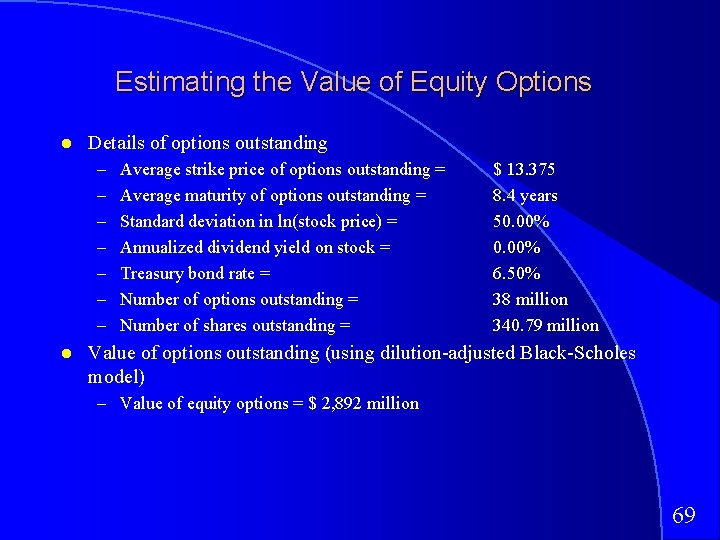Estimating the Value of Equity Options Details of options outstanding – – – – Average strike price of options outstanding = Average maturity of options outstanding = Standard deviation in ln(stock price) = Annualized dividend yield on stock = Treasury bond rate = Number of options outstanding = Number of shares outstanding = \$ 13. 375 8. 4 years 50. 00% 6. 50% 38 million 340. 79 million Value of options outstanding (using dilution-adjusted Black-Scholes model) – Value of equity options = \$ 2, 892 million 6970What do you need to break-even at \$ 84? 717273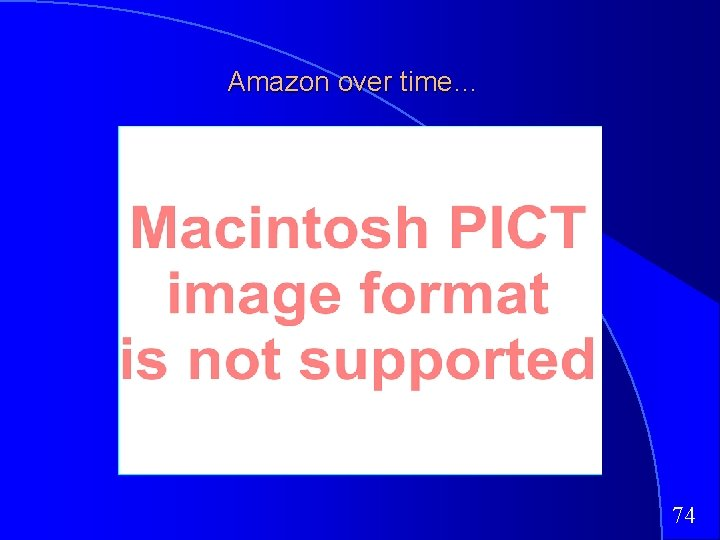Amazon over time… 74